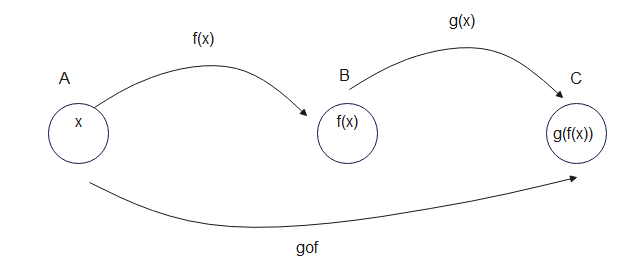# Composition of Functions

## Composition of Functions:

Let f : A-> B and g : B -> C be two functions. Then the composition of f and g, denoted by $g \circ f$, is defined as the function $g \circ f$ : A -> C given by
$g \circ f=g(f(x)$ for all x ∈ AHere's a step-by-step process to understand composition of functions
• Apply the function f(x) to the input x, and obtain the result f(x).
• Use the result f(x) as the input for the function g.
• Apply the function g to f(x) to obtain the final output, g(f(x)).
Example
f(x) =(x+3)
g(x) =x2

$g \circ f=g(f(x))=g(x+3)=(x+3)^2$
Similarly
$f \circ g=f(g(x))=f(x^2)=x^2 +3$

In this case
fog ≠ gof

## Properties of Composition of Functions

• $g \circ f$ is not necessary equal to $f \circ g$.
• Domain of $g \circ f$ is the domain of f
• if function f and g is one-one, the $g \circ f$ is one-one
• if function f and g is onto, the $g \circ f$ is onto

## Theorem Composition of three function

If f : X -> Y, g : Y -> Z and h : Z -> S are functions, then composite of these three function are
$h \circ (g \circ f)$ and $(h \circ g ) \circ f)$
Theorem
$h \circ (g \circ f) =(h \circ g ) \circ f$
Proof
$h \circ (g \circ f) (x) =h \circ (g(f(x))= h(g(f(x))$ for all x ∈ A
$(h \circ g ) \circ f (x) =h \circ g (f(x)= h(g(f(x))$ for all x ∈ A
Hence $h \circ (g \circ f) =(h \circ g ) \circ f$

## Solved Example

Example 1
Consider f : N -> N, g : N -> N and h : N -> R defined as
$f(x) = 2x$,$g(y) = 3y + 4$ and $h(z) = sin z$, for x, y and z in N.
Show that $h \circ (g \circ f ) = (h \circ g) \circ f$.
Solution
We have
$h \circ (g \circ f )$ (x) = h(gof (x)) = h(g(f (x))) = h(g(2x))
= h(3(2x) + 4) = h(6x + 4) = sin (6x + 4) for x in N.
Also,
$(h \circ g) \circ f$ (x) = (hog) ( f (x)) = (hog) (2x) = h ( g (2x))
= h(3(2x) + 4) = h(6x + 4) = sin (6x + 4), for x in N.
This shows that ho(gof) = (hog) of

Example 2
Given $f(x) = x^2 + 1$ and $g(x) = 5x +3$, find
(a) (f o g)(x)
(b) (g o f)(x)
Solution
(a) (f o g)(x)
$= f(5x+3)$
$= (5x+3)^2 + 1$
$= 25x^2 + 30x + 9 + 1$
$= 25x^2 + 30x + 10$
(b) (g o f)(x)
$= g(x^2 + 1)$
$= 5(x^2 + 1) + 3$
$= 5x^2 + 8$

Go back to Class 12 Main Page using below links